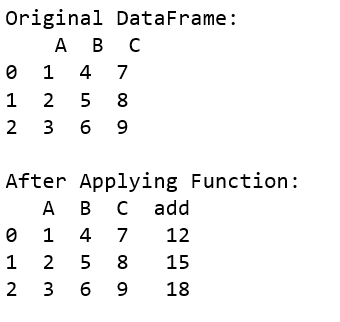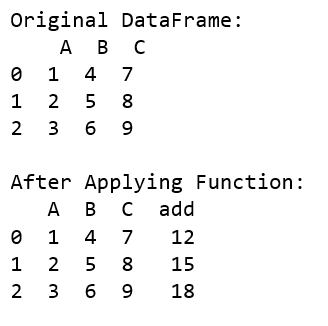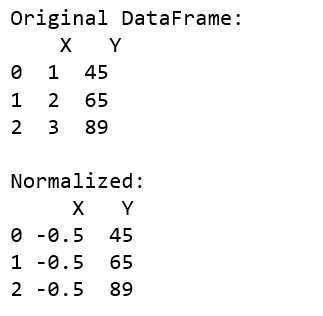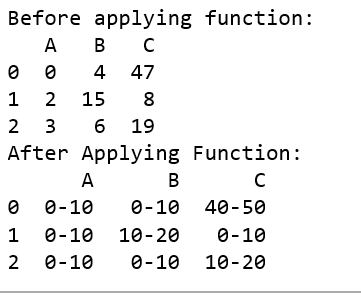# Apply function to every row in a Pandas DataFrame

Python is a great language for performing data analysis tasks. It provides with a huge amount of Classes and function which help in analyzing and manipulating data in an easier way.

One can use `apply()` function in order to apply function to every row in given dataframe. Let’s see the ways we can do this task.

Example #1:

 `# Import pandas package  ` `import` `pandas as pd ` ` `  `# Function to add ` `def` `add(a, b, c): ` `    ``return` `a ``+` `b ``+` `c ` ` `  `def` `main(): ` `     `  `    ``# create a dictionary with ` `    ``# three fields each ` `    ``data ``=` `{ ` `            ``'A'``:[``1``, ``2``, ``3``],  ` `            ``'B'``:[``4``, ``5``, ``6``],  ` `            ``'C'``:[``7``, ``8``, ``9``] } ` `     `  `    ``# Convert the dictionary into DataFrame  ` `    ``df ``=` `pd.DataFrame(data) ` `    ``print``(``"Original DataFrame:\n"``, df) ` `     `  `    ``df[``'add'``] ``=` `df.``apply``(``lambda` `row : add(row[``'A'``], ` `                     ``row[``'B'``], row[``'C'``]), axis ``=` `1``) ` `  `  `    ``print``(``'\nAfter Applying Function: '``) ` `    ``# printing the new dataframe ` `    ``print``(df) ` `  `  `if` `__name__ ``=``=` `'__main__'``: ` `    ``main() `

Output:Example #2:

You can use the `numpy` function as the parameters to the dataframe as well.

 `import` `pandas as pd ` `import` `numpy as np ` `  `  `def` `main(): ` `     `  `    ``# create a dictionary with ` `    ``# five fields each ` `    ``data ``=` `{ ` `            ``'A'``:[``1``, ``2``, ``3``],  ` `            ``'B'``:[``4``, ``5``, ``6``],  ` `            ``'C'``:[``7``, ``8``, ``9``] } ` `     `  `    ``# Convert the dictionary into DataFrame  ` `    ``df ``=` `pd.DataFrame(data) ` `    ``print``(``"Original DataFrame:\n"``, df) ` `     `  `    ``# applying function to each row in the dataframe ` `    ``# and storing result in a new coloumn ` `    ``df[``'add'``] ``=` `df.``apply``(np.``sum``, axis ``=` `1``) ` `  `  `    ``print``(``'\nAfter Applying Function: '``) ` `    ``# printing the new dataframe ` `    ``print``(df) ` `  `  `if` `__name__ ``=``=` `'__main__'``: ` `    ``main() `

Output:Example #3: Normalising Data

 `# Import pandas package  ` `import` `pandas as pd ` ` `  `def` `normalize(x, y): ` `    ``x_new ``=` `((x ``-` `np.mean([x, y])) ``/` `             ``(``max``(x, y) ``-` `min``(x, y))) ` `     `  `    ``# print(x_new) ` `    ``return` `x_new ` ` `  `def` `main(): ` `     `  `    ``# create a dictionary with three fields each ` `    ``data ``=` `{ ` `        ``'X'``:[``1``, ``2``, ``3``],  ` `        ``'Y'``:[``45``, ``65``, ``89``] } ` `     `  `    ``# Convert the dictionary into DataFrame  ` `    ``df ``=` `pd.DataFrame(data) ` `    ``print``(``"Original DataFrame:\n"``, df) ` `     `  `    ``df[``'X'``] ``=` `df.``apply``(``lambda` `row : normalize(row[``'X'``], ` `                                  ``row[``'Y'``]), axis ``=` `1``) ` `  `  `    ``print``(``'\nNormalized:'``) ` `    ``print``(df) ` `  `  `if` `__name__ ``=``=` `'__main__'``: ` `    ``main() `

Output:Example #4: Generate range

 `import` `pandas as pd ` `import` `numpy as np ` `  `  `pd.options.mode.chained_assignment ``=` `None` ` `  `# Function to generate range ` `def` `generate_range(n): ` `     `  `    ``# printing the range for eg: ` `    ``# input is 67 output is 60-70 ` `    ``n ``=` `int``(n) ` `     `  `    ``lower_limit ``=` `n``/``/``10` `*` `10` `    ``upper_limit ``=` `lower_limit ``+` `10` `     `  `    ``return` `str``(``str``(lower_limit) ``+` `'-'` `+` `str``(upper_limit)) ` `      `  `def` `replace(row): ` `    ``for` `i, item ``in` `enumerate``(row): ` `         `  `        ``# updating the value of the row ` `        ``row[i] ``=` `generate_range(item) ` `    ``return` `row ` `          `  `  `  `def` `main(): ` `    ``# create a dictionary with ` `    ``# three fields each ` `    ``data ``=` `{ ` `            ``'A'``:[``0``, ``2``, ``3``],  ` `            ``'B'``:[``4``, ``15``, ``6``],  ` `            ``'C'``:[``47``, ``8``, ``19``] } ` `     `  `    ``# Convert the dictionary into DataFrame  ` `    ``df ``=` `pd.DataFrame(data) ` `  `  `    ``print``(``'Before applying function: '``) ` `    ``print``(df) ` `      `  `    ``# applying function to each row in ` `    ``# dataframe and storing result in a new coloumn ` `    ``df ``=` `df.``apply``(``lambda` `row : replace(row)) ` `      `  `  `  `    ``print``(``'After Applying Function: '``) ` `    ``# printing the new dataframe ` `    ``print``(df) ` `  `  `if` `__name__ ``=``=` `'__main__'``: ` `    ``main() `

Output:My Personal Notes arrow_drop_upCheck out this Author's contributed articles.

If you like GeeksforGeeks and would like to contribute, you can also write an article using contribute.geeksforgeeks.org or mail your article to contribute@geeksforgeeks.org. See your article appearing on the GeeksforGeeks main page and help other Geeks.

Please Improve this article if you find anything incorrect by clicking on the "Improve Article" button below.

Article Tags :

Be the First to upvote.

Please write to us at contribute@geeksforgeeks.org to report any issue with the above content.